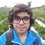# Pre-RMO 2014/2

The first term of a sequence is $2014$. Each succeeding term is the sum of the cubes of the digits of the previous term. What is the $2014^{\text{th}}$ term of the sequence?

This note is part of the set Pre-RMO 2014Note by Pranshu Gaba
6 years, 9 months ago

This discussion board is a place to discuss our Daily Challenges and the math and science related to those challenges. Explanations are more than just a solution — they should explain the steps and thinking strategies that you used to obtain the solution. Comments should further the discussion of math and science.

When posting on Brilliant:

• Use the emojis to react to an explanation, whether you're congratulating a job well done , or just really confused .
• Ask specific questions about the challenge or the steps in somebody's explanation. Well-posed questions can add a lot to the discussion, but posting "I don't understand!" doesn't help anyone.
• Try to contribute something new to the discussion, whether it is an extension, generalization or other idea related to the challenge.

MarkdownAppears as
*italics* or _italics_ italics
**bold** or __bold__ bold
- bulleted- list
• bulleted
• list
1. numbered2. list
1. numbered
2. list
Note: you must add a full line of space before and after lists for them to show up correctly
paragraph 1paragraph 2

paragraph 1

paragraph 2

[example link](https://brilliant.org)example link
> This is a quote
This is a quote
    # I indented these lines
# 4 spaces, and now they show
# up as a code block.

print "hello world"
# I indented these lines
# 4 spaces, and now they show
# up as a code block.

print "hello world"
MathAppears as
Remember to wrap math in $$ ... $$ or $ ... $ to ensure proper formatting.
2 \times 3 $2 \times 3$
2^{34} $2^{34}$
a_{i-1} $a_{i-1}$
\frac{2}{3} $\frac{2}{3}$
\sqrt{2} $\sqrt{2}$
\sum_{i=1}^3 $\sum_{i=1}^3$
\sin \theta $\sin \theta$
\boxed{123} $\boxed{123}$

Sort by:

Let's define this sequence as $a_1, a_2, a_3, ..., a_{2014}, ...$ where $a_1 = 2014$. The sum of the cubes of the digits of 2014 is 73. $a_2 = 73$. The sum of the cubes of the digits of 73 is 370. $a_3 = 370$. The sum of the cubes of the digits of 370 is 370 again. From this, we yield $a_k = a_{k+1}$ for $k \geq 3$. Therefore, $a_{2014} = a_3 = 370$. Therefore, the answer is 370.

- 6 years, 9 months ago

That is an Armstrong number. :-)

- 5 years, 9 months ago

nice

- 6 years, 9 months ago

An elegant solution.

- 6 years, 6 months ago

$1^{st}$ term $=2014$

$2^{nd}$ term $=73$

$3^{rd}$ term $=370$

$4^{th}$ term $=370$

. . .

Same goes on and $2014^{th}$ term $=\boxed{370}$

- 5 years, 11 months ago

First term is 2014,as per the given question the second term should be 2^3+1^3+4^3=73.Similarly third term will be 7^3 + 3^3 = 370.Now the rest of the terms as we go further comes out to be 370. So the ans is 370.

- 6 years, 9 months ago

370

- 6 years, 9 months ago

Here I term is 2014. Second term is (2^3+0^3+1^3+4^3)=73. Third term is (7^4+3^3)=370. Now it is clear that ii and iii digits have only two natural no. And now if we sum the cube of digits then it will remain 370. Hence after iii term all the terms of this series will be 370. Hence answer is 370.

- 6 years, 9 months ago

370

- 6 years, 9 months ago

Here 2014=2^3+1^3+4^3=73 Second term =7^3+3^3=370 Third term=3^3+7^3=370 Therefore k>=3. Then a=370

- 6 years, 9 months ago

370

- 6 years, 9 months ago

370

- 6 years, 9 months ago

370

- 6 years, 9 months ago

370

- 6 years, 9 months ago

370

- 6 years, 9 months ago

370

- 6 years, 8 months ago

370 :).

- 6 years, 7 months ago

370

- 5 years, 11 months ago

the answer is 370 because all the terms after the third term are 370

- 5 years ago

370

- 2 years, 11 months ago